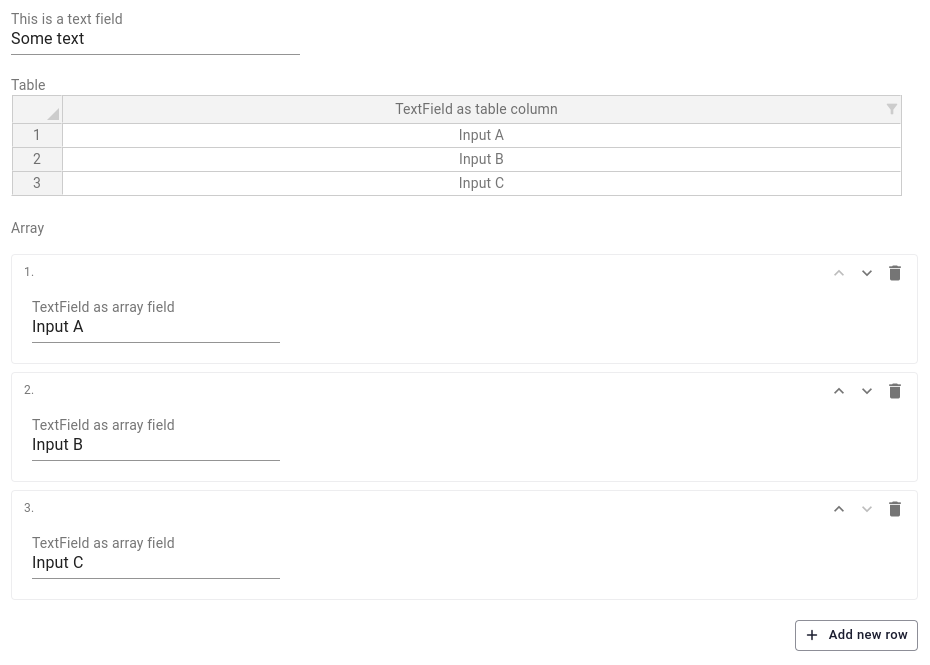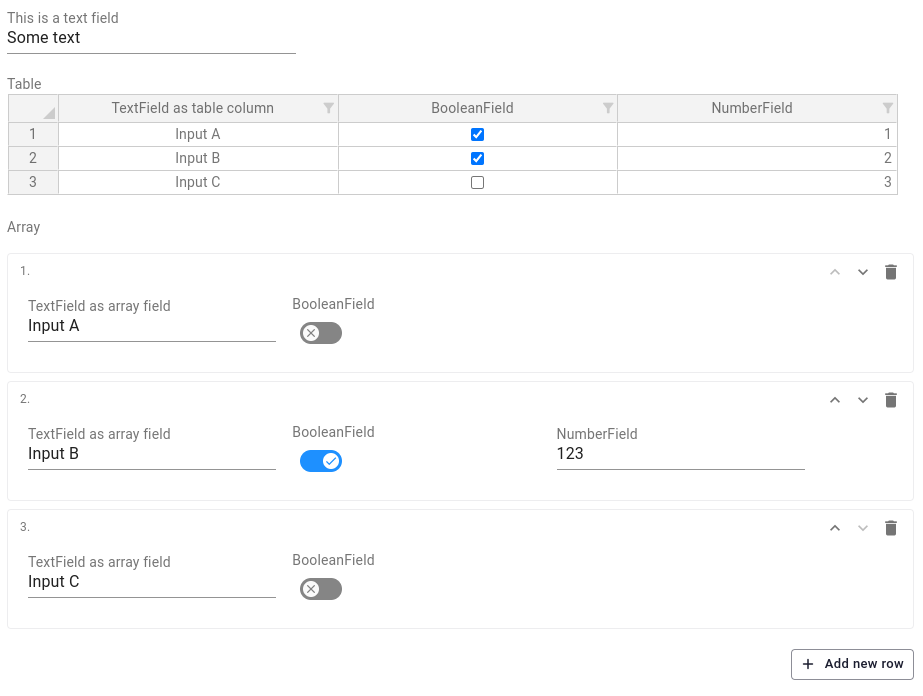# Tables & arrays

Changed in v12.1.0

`TableInput` has been renamed to `Table`

Both a `Table` and a `DynamicArray` can be used if the user must be able to make a dynamic number of objects sharing the same properties. The similarities and differences between the two are discussed below.Table (top) and DynamicArray (bottom)

## Table​

Set up a `Table` and add fields using the dot-notation as follows:

``from viktor.parametrization import ..., Tableclass Parametrization(ViktorParametrization):    table = Table('Table')    table.col_1 = TextField('TextField as table column')``

## DynamicArray​

Set up a `DynamicArray` and add fields using the dot-notation as follows:

``from viktor.parametrization import ..., DynamicArrayclass Parametrization(ViktorParametrization):    array = DynamicArray('Array')    array.col_1 = TextField('TextField as array field')``

## Differences Table vs. DynamicArray​

In addition to visual difference, there are more practical differences between the input fields which may determine the best solution for your use-case. The following comparison can be made:

TableDynamicArray
CompactLess compact
RectangularNot necessarily rectangular
Defaults when adding a new row is not supportedDefaults when adding a new row is supported
Options in OptionField cannot make use of a `label`Options in OptionField can make use of a `label`
Supports copy-paste of complete table contentSupports copy-paste per input field only
User can create rowsUser can create rows
User cannot create columnsUser cannot create fields, but may use dynamic visibility

Furthermore, not all regular fields can be used in both of these multi-row fields. The table below shows which fields are supported in the `Table` and `DynamicArray`

FieldTableDynamicArray
Text××
TextField
TextAreaField×
NumberField
IntegerField
BooleanField
DateField×
OutputField××
HiddenField××
LineBreak×
OptionField
MultiSelectField×
AutocompleteField
EntityOptionField×
ChildEntityOptionField×
SiblingEntityOptionField×
EntityMultiSelectField×
ChildEntityMultiSelectField×
SiblingEntityMultiSelectField×
FileField×
MultiFileField×
GeoPointField×✓ (>= v12.2.0)
GeoPolylineField×✓ (>= v12.2.0)
GeoPolygonField×✓ (>= v12.2.0)
ColorField×✓ (>= v14.0.0)
ActionButton××
In the comparison, rectangular means that each row in the `Table` contains the same columns visually as well as in the parameter set. Rows in a `DynamicArray` on the other hand could contain different input fields, by hiding certain fields when, for example, the user triggers a toggle.
``from viktor.parametrization import ViktorParametrization, DynamicArray, Table, RowLookup, NumberField, TextField, \    BooleanFieldclass Parametrization(ViktorParametrization):    field = TextField('This is an example field')    # table    table = Table('Table')    table.col_1 = TextField('TextField as table column')    table.col_2 = BooleanField('BooleanField')    table.col_3 = NumberField('NumberField')  # visible not supported    # dynamic array    array = DynamicArray('Array')    array.col_1 = TextField('TextField as array field')    array.col_2 = BooleanField('BooleanField')    array.col_3 = NumberField('NumberField', visible=RowLookup('col_2'))``
In this example, a `DynamicArray` row in which the toggle (`col_2`) is active will also show the NumberField (`col_3`). In a `Table` `col_3` is always visible.Visibility of columns/fields